contour

Contour plot of matrix

•Description

example

contour(Z) creates a contour plot containing the isolines of matrix Z, where Z contains height values on the x-y plane. MATLAB® automatically selects the contour lines to display. The column and row indices of Z are the x and y coordinates in the plane, respectively.

example

contour(X,Y,Z) specifies the x and y coordinates for the values in Z.

example

contour(___,levels) specifies the contour lines to display as the last argument in any of the previous syntaxes. Specify levels as a scalar value n to display the contour lines at n automatically chosen levels (heights). To draw the contour lines at specific heights, specify levels as a vector of monotonically increasing values. To draw the contours at one height (k), specify levels as a two-element row vector [k k].

example

contour(___,LineSpec) specifies the style and color of the contour lines.

example

contour(___,Name,Value) specifies additional options for the contour plot using one or more name-value pair arguments. Specify the options after all other input arguments. For a list of properties, see Contour Properties.

contour(ax,___) displays the contour plot in the target axes. Specify the axes as the first argument in any of the previous syntaxes.

M = contour(___) returns the contour matrix M, which contains the (x, y) coordinates of the vertices at each level.

example

[M,c] = contour(___) returns the contour matrix and the contour object c. Use c to set properties after displaying the contour plot.

Examples

collapse all

Create matrices X and Y, that define a grid in the x-y plane. Define matrix Z as the heights above that grid. Then plot the contours of Z.

x = linspace(-2*pi,2*pi);
y = linspace(0,4*pi);
[X,Y] = meshgrid(x,y);
Z = sin(X)+cos(Y);
contour(X,Y,Z)Define Z as a function of X and Y. In this case, call the peaks function to create X, Y, and Z. Then plot 20 contours of Z.

[X,Y,Z] = peaks;
contour(X,Y,Z,20)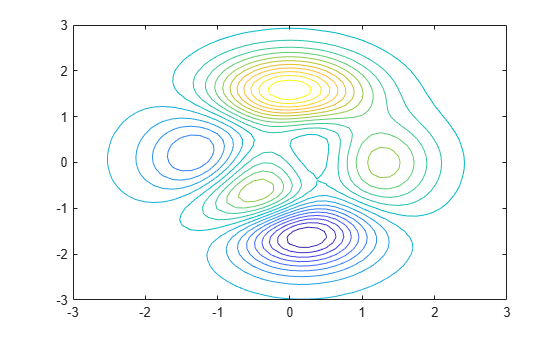Display the contours of the peaks function at Z = 1.

[X,Y,Z] = peaks;
v = [1,1];
contour(X,Y,Z,v)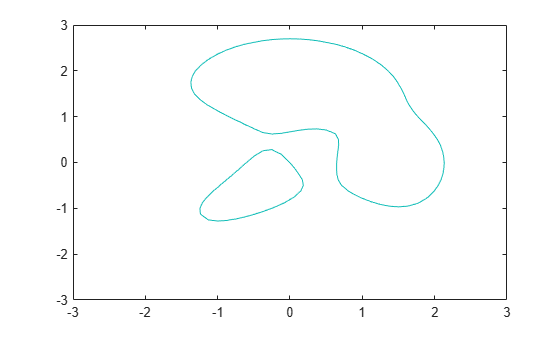Create a contour plot of the peaks function, and specify the dashed line style.

[X,Y,Z] = peaks;
contour(X,Y,Z,'--')Define Z as a function of two variables, X and Y. Then create a contour plot of that function, and display the labels by setting the ShowText property to 'on'.

x = -2:0.2:2;
y = -2:0.2:3;
[X,Y] = meshgrid(x,y);
Z = X.*exp(-X.^2-Y.^2);
contour(X,Y,Z,'ShowText','on')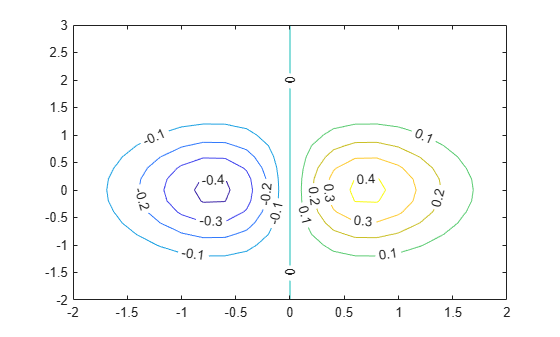Create a contour plot of the peaks function. Make the contour lines thicker by setting the LineWidth property to 3.

Z = peaks;
[M,c] = contour(Z);
c.LineWidth = 3;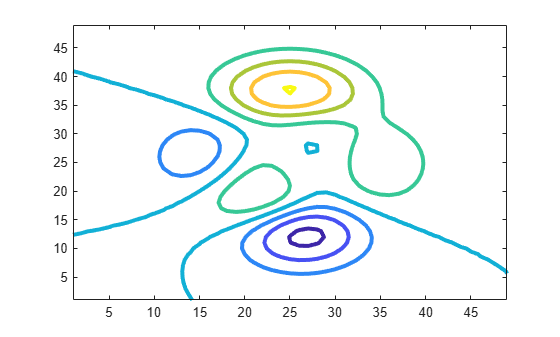Insert NaN values wherever there are discontinuities on a surface. The contour function does not draw contour lines in those regions.

Define matrix Z as a sampling of the peaks function. Replace all values in column 26 with NaN values. Then plot the contours of the modified Z matrix.

Z = peaks;
Z(:,26) = NaN;
contour(Z)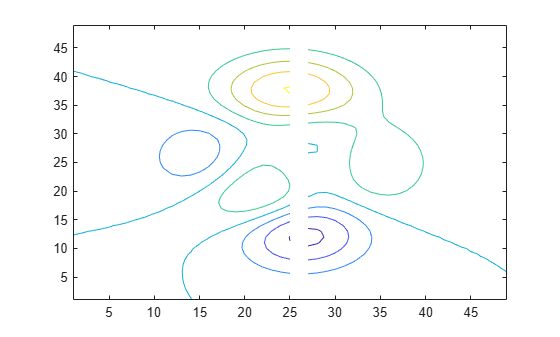Input Arguments

collapse all

x-coordinates, specified as a matrix the same size as Z, or as a vector with length n, where [m,n] = size(Z). The default value of X is the vector (1:n).

When X is a matrix, the values must be strictly increasing or decreasing along one dimension and remain constant along the other dimension. The dimension that varies must be the opposite of the dimension that varies in Y. You can use the meshgrid function to create X and Y matrices.

When X is a vector, the values must be strictly increasing or decreasing.

Example: X = 1:10

Example: X = [1 2 3; 1 2 3; 1 2 3]

Example: [X,Y] = meshgrid(1:10)

The XData property of the Contour object stores the x-coordinates.

Data Types: single | double | int8 | int16 | int32 | int64 | uint8 | uint16 | uint32 | uint64

y-coordinates, specified as a matrix the same size as Z, or as a vector with length m, where [m,n] = size(Z). The default value of Y is the vector (1:m).

When Y is a matrix, the values must be strictly increasing or decreasing along one dimension and remain constant along the other dimension. The dimension that varies must be the opposite of the dimension that varies in X. You can use the meshgrid function to create the X and Y matrices.

When Y is a vector, the values must be strictly increasing or decreasing.

Example: Y = 1:10

Example: Y = [1 1 1; 2 2 2; 3 3 3]

Example: [X,Y] = meshgrid(1:10)

The YData property of the Contour object stores the y-coordinates.

Data Types: single | double | int8 | int16 | int32 | int64 | uint8 | uint16 | uint32 | uint64

z-coordinates, specified as a matrix. This matrix must have at least two rows and two columns, and it must contain at least two different values.

Example: Z = peaks(20)

The ZData property of the Contour object stores the z-coordinates.

Data Types: single | double | int8 | int16 | int32 | int64 | uint8 | uint16 | uint32 | uint64

Contour levels, specified as a scalar whole number or a vector. Use this argument to control the number and location of the contour lines. When you do not specify the levels, the contour function chooses the levels automatically.

• To draw contour lines at n automatically chosen heights, specify levels as the scalar value n.

• To draw the contour lines at specific heights, specify levels as a vector of monotonically increasing values.

• To draw contour lines at a single height k, specify levels as a two-element row vector [k k].

Example: contour(peaks,10) draws contour lines at 10 automatically chosen heights on the peaks function.

Example: contour(peaks,[-4 0 4]) draws contour lines at 3 specific heights on the peaks function: -4, 0, and 4.

Example: contour(peaks,[3 3]) draws contour lines to show where the height of the peaks function is 3.

Data Types: single | double | int8 | int16 | int32 | int64 | uint8 | uint16 | uint32 | uint64

Line style and color, specified as a character vector or string scalar containing characters and symbols. The characters and symbols can appear in any order. You can specify the line style, line color, or both. Marker symbols such as 'o' are ignored.

Example: '--g' is a green dashed line.

Line StyleDescriptionResulting Line
'-'Solid line'--'Dashed line':'Dotted line'-.'Dash-dotted lineColor NameShort NameAppearance
'red''r''green''g''blue''b''cyan' 'c''magenta''m''yellow''y''black''k''white''w'Target axes, specified as an Axes object. If you do not specify the axes, then contour plots into the current axes.

Name-Value Arguments

Specify optional comma-separated pairs of Name,Value arguments. Name is the argument name and Value is the corresponding value. Name must appear inside quotes. You can specify several name and value pair arguments in any order as Name1,Value1,...,NameN,ValueN.

Example: contour(Z,'ShowText','on') displays the contour line labels.

Note

The properties listed here are only a subset. For a complete list, see Contour Properties.

Contour line labels, specified as 'on' or 'off', or as numeric or logical 1 (true) or 0 (false). A value of 'on' is equivalent to true, and 'off' is equivalent to false. Thus, you can use the value of this property as a logical value. The value is stored as an on/off logical value of type matlab.lang.OnOffSwitchState.

• 'on' — Display the height values along the contour lines.

• 'off' — Do not label the contour lines.

Contour line width, specified as a positive value in points. One point equals 1/72 inch.

Label spacing along the contour lines, specified as a scalar value in points, where one point is 1/72 inch. Use this property to control the number of contour labels along the contour lines. Smaller values produce more labels.

You must set the ShowText property to 'on' for the LabelSpacing property to have an effect.

If you use the clabel function to display the labels, then the LabelSpacing property has no effect and the plot displays one label per line.

Output Arguments

collapse all

Contour matrix, returned as two-row matrix. This matrix contains the contour levels (heights) and the coordinates of the vertices at each level. The data is arranged sequentially in n sets of columns for n contour lines:

• The first column in each set contains the contour level and the number of vertices at that level. The top number is the contour level, and the bottom number is the number of vertices.

• Subsequent columns in the set are the (x, y) coordinates of the vertices. Each column represents an ordered pair. The top number is the x-coordinate, and the bottom number is the y-coordinate.

For example, here are the first few columns of the contour matrix M = contour(peaks(3)):The ContourMatrix property of the Contour object stores the contour matrix.

Contour object. Use this object to set properties after displaying the contour plot.